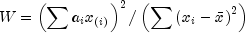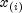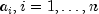JMSLTM Numerical Library 7.2.0
com.imsl.stat

## Class NormalityTest

• All Implemented Interfaces:
Serializable, Cloneable

```public class NormalityTest
extends Object
implements Serializable, Cloneable```
Performs a test for normality.

Three methods are provided for testing normality: the Shapiro-Wilk W test, the Lilliefors test, and the chi-squared test.

Shapiro-Wilk W Test

The Shapiro-Wilk W test is thought by D'Agostino and Stevens (1986, p. 406) to be one of the best omnibus tests of normality. The function is based on the approximations and code given by Royston (1982a, b, c). It can be used in samples as large as 2,000 or as small as 3. In the Shapiro and Wilk test, W is given bywhereis the i-th largest order statistic and x is the sample mean. Royston (1982) gives approximations and tabled values that can be used to compute the coefficients, and obtains the significance level of the W statistic.

Lilliefors Test

This function computes Lilliefors test and its p-values for a normal distribution in which both the mean and variance are estimated. The one-sample, two-sided Kolmogorov-Smirnov statistic D is first computed. The p-values are then computed using an analytic approximation given by Dallal and Wilkinson (1986). Because Dallal and Wilkinson give approximations in the range (0.01, 0.10) if the computed probability of a greater D is less than 0.01, the p-value is set to 0.50. Note that because parameters are estimated, p-values in Lilliefors test are not the same as in the Kolmogorov-Smirnov Test.

Observations should not be tied. If tied observations are found, an informational message is printed. A general reference for the Lilliefors test is Conover (1980). The original reference for the test for normality is Lilliefors (1967).

Chi-Squared Test

This function computes the chi-squared statistic, its p-value, and the degrees of freedom of the test. Argument n finds the number of intervals into which the observations are to be divided. The intervals are equiprobable except for the first and last interval, which are infinite in length.

If more flexibility is desired for the specification of intervals, the same test can be performed with class `ChiSquaredTest`.

Example, Serialized Form
• ### Nested Class Summary

Nested Classes
Modifier and Type Class and Description
`static class ` `NormalityTest.NoVariationInputException`
There is no variation in the input data.
• ### Constructor Summary

Constructors
Constructor and Description
`NormalityTest(double[] x)`
Constructor for `NormalityTest`.
• ### Method Summary

Methods
Modifier and Type Method and Description
`double` `ChiSquaredTest(int n)`
Performs the chi-squared goodness-of-fit test.
`double` `getChiSquared()`
Returns the chi-square statistic for the chi-squared goodness-of-fit test.
`double` `getDegreesOfFreedom()`
Returns the degrees of freedom for the chi-squared goodness-of-fit test.
`double` `getMaxDifference()`
Returns the maximum absolute difference between the empirical and the theoretical distributions for the Lilliefors test.
`double` `getShapiroWilkW()`
Returns the Shapiro-Wilk W statistic for the Shapiro-Wilk W test.
`double` `LillieforsTest()`
Performs the Lilliefors test.
`double` `ShapiroWilkWTest()`
Performs the Shapiro-Wilk W test.
• ### Methods inherited from class java.lang.Object

`clone, equals, finalize, getClass, hashCode, notify, notifyAll, toString, wait, wait, wait`
• ### Constructor Detail

• #### NormalityTest

`public NormalityTest(double[] x)`
Constructor for `NormalityTest`.
Parameters:
`x` - A `double` array containing the observations. `x.length` must be in the range from 3 to 2,000, inclusive, for the Shapiro-Wilk W test and must be greater than 4 for the Lilliefors test.
• ### Method Detail

• #### ChiSquaredTest

```public final double ChiSquaredTest(int n)
throws NormalityTest.NoVariationInputException,
InverseCdf.DidNotConvergeException```
Performs the chi-squared goodness-of-fit test.
Parameters:
`n` - An `int` scalar containing the number of cells into which the observations are to be tallied.
Returns:
A `double` scalar containing the p-value for the chi-squared goodness-of-fit test.
Throws:
`NormalityTest.NoVariationInputException` - is thrown if there is no variation in the input data.
`DidNotConvergeException` - is thrown if the iteration did not converge.
`InverseCdf.DidNotConvergeException`
`ChiSquaredTest`
• #### getChiSquared

`public double getChiSquared()`
Returns the chi-square statistic for the chi-squared goodness-of-fit test.
Returns:
A `double` scalar containing the chi-square statistic. Returns `Double.NaN` for other tests.
• #### getDegreesOfFreedom

`public double getDegreesOfFreedom()`
Returns the degrees of freedom for the chi-squared goodness-of-fit test.
Returns:
A `double` scalar containing the degrees of freedom. Returns `Double.NaN` for other tests.
• #### getMaxDifference

`public double getMaxDifference()`
Returns the maximum absolute difference between the empirical and the theoretical distributions for the Lilliefors test.
Returns:
A `double` scalar containing the maximum absolute difference between the empirical and the theoretical distributions. Returns `Double.NaN` for other tests.
• #### getShapiroWilkW

`public double getShapiroWilkW()`
Returns the Shapiro-Wilk W statistic for the Shapiro-Wilk W test.
Returns:
A `double` scalar containing the Shapiro-Wilk W statistic. Returns `Double.NaN` for other tests.
• #### LillieforsTest

```public final double LillieforsTest()
throws NormalityTest.NoVariationInputException,
InverseCdf.DidNotConvergeException```
Performs the Lilliefors test.
Returns:
A `double` scalar containing the p-value for the Lilliefors test. Probabilities less than 0.01 are reported as 0.01, and probabilities greater than 0.10 for the normal distribution are reported as 0.5. Otherwise, an approximate probability is computed.
Throws:
`NormalityTest.NoVariationInputException` - is thrown if there is no variation in the input data.
`DidNotConvergeException` - is thrown if the iteration did not converge.
`InverseCdf.DidNotConvergeException`
• #### ShapiroWilkWTest

```public final double ShapiroWilkWTest()
throws NormalityTest.NoVariationInputException,
InverseCdf.DidNotConvergeException```
Performs the Shapiro-Wilk W test.
Returns:
A `double` scalar containing the p-value for the Shapiro-Wilk W test.
Throws:
`NormalityTest.NoVariationInputException` - is thrown if there is no variation in the input data.
`DidNotConvergeException` - is thrown if the iteration did not converge.
`InverseCdf.DidNotConvergeException`
JMSLTM Numerical Library 7.2.0